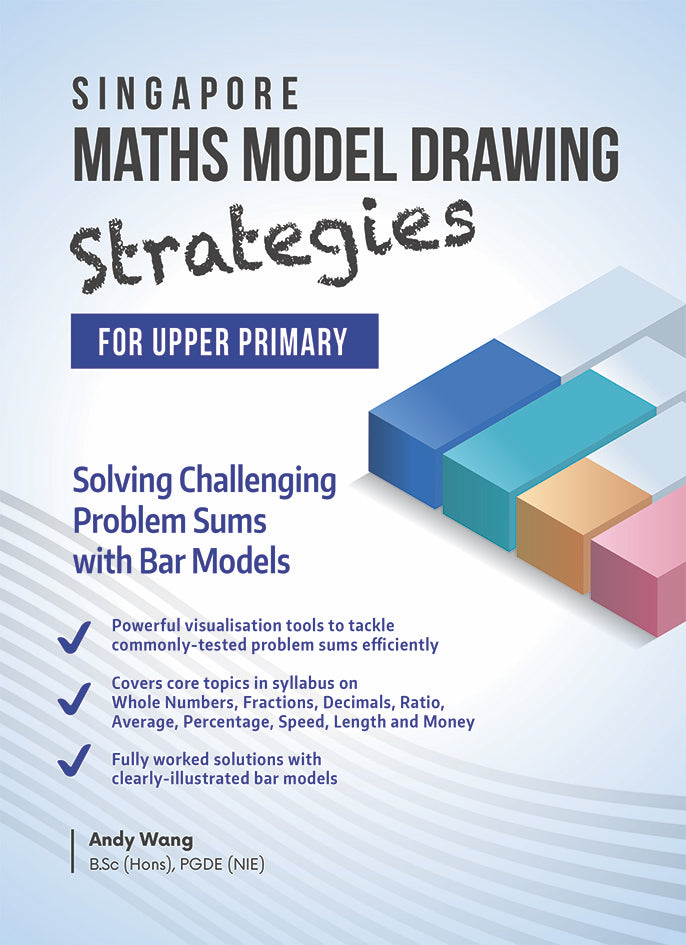1/ 6

# Singapore Maths Model Drawing Strategies for Upper Primary

\$963
\$000
Quantity
Only 1 left
Secure payments

The Singapore Mathematics Model Drawing Method is a mathematical concept that is used to teach problem-solving skills among primary school students. It is great for children who are visual learners as it gives them a better understanding of the questions that they face in mathematics examinations. Using blocks to represent numbers is more visually appealing to them than algebra, which uses alphabets instead. By visualising the question, children will be able to see the logic and the relationships between different objects.

The questions in this book are designed to enable progressive learning. There are step-by-step solutions to ensure students master the skill of model drawing in various core topics of the primary school mathematics syllabus such as whole numbers, fractions, decimals, ratio, average, percentage, speed, length, money, mass and volume.

The following model drawing concepts will be acquired by students who have conscientiously completed this book:

• Basic Level Model Concepts:
Basic Comparison Model Concept
Basic Units Model Concept
Basic Equalisation Model Concept
Basic Transfer Model Concept
• Intermediate Level Model Concepts:
Remainder Model Concept